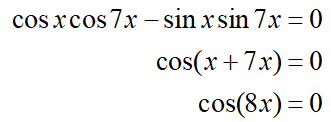# use an addition or subtraction formula to simplify the following equation, then find all solutions in the interval [0. radian/4].equation: cosx cos7x-sinx sin7x=0its an addition formula for cosine, so simplified it would be cos(8x)finding all solutions, and putting them in interval form is what I am confused with

Question
1 views

use an addition or subtraction formula to simplify the following equation, then find all solutions in the interval [0. radian/4].

equation: cosx cos7x-sinx sin7x=0

its an addition formula for cosine, so simplified it would be cos(8x)

finding all solutions, and putting them in interval form is what I am confused with

check_circle

Step 1

The addition formula for cosine is given by

cos(a + b) = cos a cos b – sin a sin b

Step 2

Use the addition formula for cosine and simplify the given equation as follows....

### Want to see the full answer?

See Solution

#### Want to see this answer and more?

Solutions are written by subject experts who are available 24/7. Questions are typically answered within 1 hour.*

See Solution
*Response times may vary by subject and question.
Tagged in